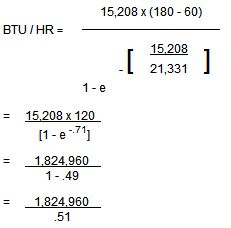# Calculating Boiler Size for Tank Rubber Lining Steam Curing

Blair tank rubber lining experts explain how to calculate the boiler size when steam curing rubber linings. Blair Rubber’s technical staff is available to provide further assistance with Blair’s products and questions/answers that are not addressed in the following bulletin.

Calculation for Tank Size for Steam CuringHeat loss is expressed as BTU’s lost per square foot per degree Fahrenheit above ambient.

TF is the final temperature you expect to achieve.

TI is the initial or ambient temperature.

e is the natural logarithm.

Specific heat is expressed as BTU’s required to raise the temperatures of one pound of material one degree Fahrenheit in one hour.

This formula uses heat loss and specific heat of the entire tank.

You will need the area of the tank in square feet for the calculations.

To calculate heat loss of a tank sitting on a concrete pad, multiply the area of the roof and sides by 2.4 if un-insulated and by .5 if insulated. Add this to the area of the bottom multiplied by .1 and you have the heat loss of the tank. If the tank is elevated, use the same multiplier for the bottom that you used for the roof and sides.

To calculate specific heat, you will need the total weight of the tank and 4 inches of concrete under the tank. 1/8 inch steel weighs 5.1 pounds per square foot. Concrete weighs 140 pounds per cubic foot. For open top tanks, assume 1/4 inch thick steel. Multiply the total weight by .14 and you have the specific heat of the system. If the tank is elevated, use only the weight of the steel.

As an example, I’ll calculate the boiler required to cure a 40 foot diameter by 40 foot high open top tank on a concrete pad. The average thickness of the walls is 5/16 inch thick, the floor is 3/8 inch and I assume 1/4 inch thick steel for the open top. Ambient temperature is 60 degrees Fahrenheit and final temperature is 180 degrees Fahrenheit.

Area of the roof = 1257 square feet

Area of the walls = 5027 square feet

Area of the floor = 1257 square feet

The volume of concrete = .33 feet x 1257 square feet = 415 cubic feet

Calculate heat loss – un-insulated

1257 FT2 + 5027 FT2 = 6284 FT2

6284 x 2.4 = 15,082 BTU/HR/°F

1257 FT2 x .1 = 126 BTU/HR/°F

Total heat loss = 15,208 BTU/HR/°F

Calculate specific heat

Roof = 1257 FT2 x 10 lbs = 12,570 lbs

Walls = 5027 FT2 x 12.5 lbs = 62,838 lbs

Floor = 1257 FT2 x 15 lbs = 18,855 lbs

Concrete = 415 FT3 x 140 lbs = 58,100 lbs

Total weight = 152,363 lbs

Specific heat = 152,363 x .14 = 21,331

You now have all the information to calculate boiler size.BTU / HR = 3,578,353

1 boiler horsepower = 33,500 BTU / HR

3,578,353 x 33,500 = 107 horsepower

A 100 horsepower boiler will work but, would prefer to use a 125 horsepower boiler.

The same tank with insulation calculates to 84 horsepower. The preference would be a 100 horsepower boiler.

Generally, you should increase the boiler size for open top tanks because it is impossible to prevent steam leaking around your cover.